Ethereum Classic Blog

# Ethereum Classic Recursive Length Prefix Encoding

Christian SeberinoEthereum Classic (ETC) uses Recursive Length Prefix (RLP) encoding to store and send data structures. I will describe RLP and how it is used in ETC.

## .css-1r8a28j{-webkit-text-decoration:none!important;text-decoration:none!important;}.css-1r8a28j:hover{-webkit-text-decoration:underline!important;text-decoration:underline!important;}Introduction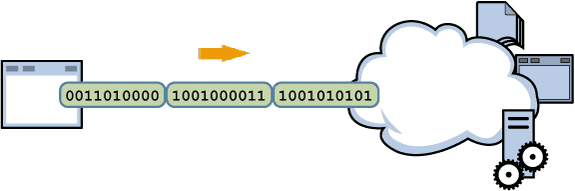Serialization is the process of encoding data structures into byte sequences. It is also referred to as marshalling and pickling. Serialization is necessary when storing and sending data structures.

RLP is a serialization format created by Ethereum developers for all data structures such as accounts, transactions and blocks. RLP is simpler than the alternatives such as Extensible Markup Language (XML), JavaScript Object Notation (JSON), Binary JSON (BSON), Protocol Buffers and Bencode.

RLP is also consistent. Identical objects are always converted to identical byte sequences. This is not true of all serialization formats. For example, when encoding sets of key value pairs, some schemes do not specify an ordering for the keys.

## DetailsRLP operates on byte sequences and lists. Lists can contain byte sequences and other lists. The interpretation of all inputs is handled by other protocols. For byte sequences, small headers are added which depend on the length. For lists, the elements are encoded separately and concatenated. As with byte sequences, small headers are added which depend on the length. Lastly, all lengths are encoded in big endian format.

## CodeHere are Python functions which implement RLP encoding and decoding:

``````#!/usr/bin/env python3

import math

N_BITS_PER_BYTE = 8

def n_bytes(integer):
"""
Finds the numbers of bytes needed to represent integers.
"""

return math.ceil(integer.bit_length() / N_BITS_PER_BYTE)

def get_len(input, extra):
"""
Finds the lengths of the longest inputs using the given extra values.
"""

n_bytes = input - extra

return 1 + n_bytes + int.from_bytes(input[2:2 + n_bytes], "big")

def rlp_encode(input):
"""
Recursive Length Prefix encodes inputs.
"""

if isinstance(input, bytes):
body = input
if   (len(body) == 1) and (body < 128):
elif len(body) < 56:
else:
len_   = len(body)
len_   = len_.to_bytes(n_bytes(len_), "big")
header = bytes([len(len_) + 183]) + len_
else:
body = bytes([])
for e in input:
body += rlp_encode(e)
if len(body) < 56:
else:
len_   = len(body)
len_   = len_.to_bytes(n_bytes(len_), "big")
header = bytes([len(len_) + 247]) + len_

return result

def rlp_decode(input):
"""
Recursive Length Prefix decodes inputs.
"""

if   input < 128:
result = input
elif input < 184:
result = input[1:]
elif input < 192:
result = input[1 + (input - 183):]
else:
result = []
if input < 248:
input = input[1:]
else:
input = input[1 + (input - 247):]
while input:
if   input < 128:
len_ = 1
elif input < 184:
len_ = 1 + (input - 128)
elif input < 192:
len_ = get_len(input, 183)
elif input < 248:
len_ = 1 + (input - 192)
else:
len_ = get_len(input, 247)
result.append(rlp_decode(input[:len_]))
input = input[len_:]

return result
``````

Notice that the functions are recursive. Notice also that the functions work for inputs requiring up to about 18 million terabytes. Here are examples of their usage:
``````>>> rlp_encode(b"A")
b'A'

>>> rlp_encode(b"12345")
b'\x8512345'

>>> rlp_encode(20 * b"12345")
b'\xb8d1234512345123451234512345123451234512345123451234512345123451234512345123451234512345123451234512345'

>>> rlp_encode([b"12345"])
b'\xc6\x8512345'

>>> rlp_encode([b"abcde", 3 * [b"12345"], [b"fghij"], b"67890", 4 * [b"klmno"]])
b'\xf8\x85abcde\xd2\x8512345\x8512345\x8512345\xc6\x85fghij\x8567890\xd8\x85klmno\x85klmno\x85klmno\x85klmno'

>>> rlp_decode(b"\x8512345")
b'12345'

>>> rlp_decode(b"\xc6\x8512345")
[b'12345']

>>> rlp_decode(b"\xf8\x85abcde\xd2\x8512345\x8512345\x8512345\xc6\x85fghij\x8567890\xd8\x85klmno\x85klmno\x85klmno\x85klmno")
[b'abcde', [b'12345', b'12345', b'12345'], [b'fghij'], b'67890', [b'klmno', b'klmno', b'klmno', b'klmno']]
``````

## ConclusionRLP is an elegant and approachable serialization format used extensively by ETC. It can be quickly mastered thereby illuminating this important aspect of the system.

## Feedback

You can contact me by clicking any of these icons: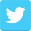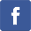## Acknowledgements

I would like to thank IOHK (Input Output Hong Kong) for funding this effort.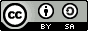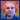cseberino
•English
•中文
•Deutsch
•Español
•Français
•Ελληνικά
•हिन्दी
•Hrvatski
•Malay
•Italiano
•日本語
•한국어
•Nederlands
•русский
•اَلْعَرَبِيَّةُ
•ภาษาไทย
•Türk
•Tiếng Việt
The ETC community is active on Discord
Discord
Discord
ETC Coop Discord
ETC Coop Discord
Github
Github
ETC Labs Github
ETC Labs Github
Reddit
Reddit
Made with `<3` for the Original Ethereum Vision